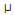# Electronics - Alternating Current vs Direct Current

### Exercise :: Alternating Current vs Direct Current - True or False

1.

DC current flows in one direction, while ac current rises from zero to a maximum, then falls to zero in one direction, then rises to a maximum, then falls to zero in the opposite direction.

 A. True B. False

Explanation:

No answer description available for this question. Let us discuss.

2.

A square and a rectangular waveform appear to have a step voltage transition.

 A. True B. False

Explanation:

No answer description available for this question. Let us discuss.

3.

The duty cycle of a square wave with a pulse width of 3s and a period of 9s is 40%.

 A. True B. False

Explanation:

No answer description available for this question. Let us discuss.

4.

The rms value of a sine wave is .707 x peak-to-peak value/2.

 A. True B. False

Explanation:

No answer description available for this question. Let us discuss.

5.

DC current transfers better over longer distances than ac current.

 A. True B. False